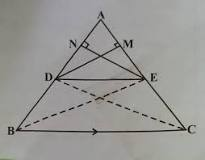Wellcome!

Nishant Verma
Pythagorean Theorem. In a right triangle, the square of the hypotenuse is equal to the sum of the squares of the other two sides.

-Answered by Nishant Verma On 30 August 2019:03:56:50 PM

Nishant Verma
If in two trianglessides of one triangle are proportional to the sides of the other trianglethen their corresponding angles are equal and hence the two triangles are similar. This is also called SSS (Side-Side-Sidecriterion.

-Answered by Nishant Verma On 30 August 2019:03:57:22 PM

Question 3. Asked on :30 August 2019:03:11:19 PM

If a line divides any of the two sides of a triangle in the same ratio, then that line is parallel to the third side.

-Added by Prince Rawat Mathematics » Triangle

Nishant Verma
If a line divides any two sides of a triangle in the same ratio,then the line is parallel to the third side.

-Answered by Nishant Verma On 30 August 2019:03:57:52 PM

Question 4. Asked on :21 August 2019:09:36:36 AM

In a right triangle the square of the hypotenuse is equal to the sum of the squares of the other two sides.

-Added by Prince Rawat Mathematics » Triangle

Suraj Kumar
Theorem 6.8 : In a right triangle, the square of the hypotenuse is equal to the sum of the squares of the other two sides.

-Answered by Suraj Kumar On 11 December 2019:08:33:38 AM

Suraj Kumar
If a line is drawn parallel to one side of a triangle to intersect the other two sides in distinct points ,then theother two sides are divided in the same ratio

.-Answered by Suraj Kumar On 11 December 2019:08:33:46 AM

You can see here all the solutions of this question by various user for NCERT Solutions. We hope this try will help you in your study and performance.

This Solution may be usefull for your practice and CBSE Exams or All label exams of secondory examination. These solutions or answers are user based solution which may be or not may be by expert but you have to use this at your own understanding of your syllabus.

What do you have in your Mind....

* Now You can earn points on every asked question and Answer by you. This points make you a valuable user on this forum. This facility is only available for registered user and educators.

Do you have a question to ask?

User Earned Point: Select

All Tags by Subjects:

Science (2235)
History (278)
Geography (310)
Economics (258)
Political Science (96)
Mathematics (201)
General Knowledge (5686)
Biology (94)
Physical Education (20)
Chemistry (118)
Civics (114)
Home Science (12)
Sociology (9)
Hindi (45)
English (259)
Physics (1435)
Other (97)
Accountancy (378)